Trigonometry : Identities of Squared Trigonometric Functions

Example Questions

Example Question #1 : Identities Of Squared Trigonometric Functions

Using trigonometric identities prove whether the following is valid: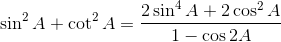False

True

Uncertain

Only in the range of: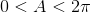Only in the range of: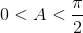True

Explanation:

We can work with either side of the equation as we choose. We work with the right hand side of the equation since there is an obvious double angle here. We can factor the numerator to receive the following: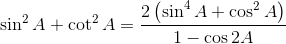Next we note the power reducing formula for sine so we can extract the necessary components as follows: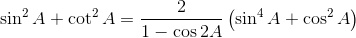The power reducing formula must be inverted giving: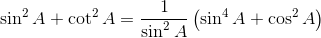Now we can distribute and reduce: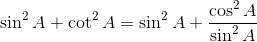Finally recalling the basic identity for the cotangent: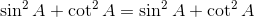This proves the equivalence.

Example Question #2 : Identities Of Squared Trigonometric Functions

Use the power reducing formulas for trigonometric functions to reduce and simplify the following equation: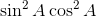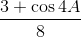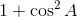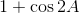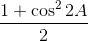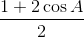Explanation:

The power reducing formulas for both sine and cosine differ in only the operation in the numerator. Applying the power reducing formulas here we get: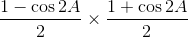Multiplying the binomials in the numerator and multiplying the denominators: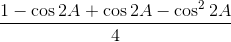Reducing the numerator: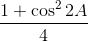We again use the power reducing formula for cosine as follows: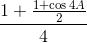Combining the numerator by determining a common denominator: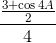Now simply reducing the double fraction:Example Question #3 : Identities Of Squared Trigonometric Functions

Using trigonometric identities, determine whether the following is valid: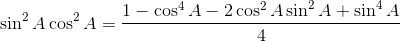Only valid in the range of:False

Uncertain

True

Only valid in the range of:False

Explanation:

In this case we choose to work with the side that appears to be simpler, the left hand side. We begin by using the power reducing formulas: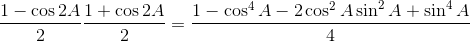Next we perform the multiplication on the numerator: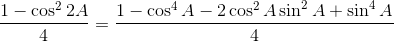The next step we take is to remove the double angle, since there is no double angle in the alleged solution: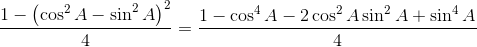Finally we multiply the binomials in the numerator on the left hand side to determine if the equivalence holds: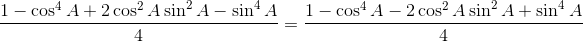We see that the equivalence does not hold.

Example Question #1 : Trigonometric Identities

Simplify the expression: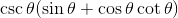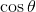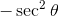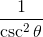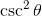1Explanation:

The first step in solving this equation is to distribute :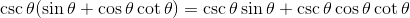At this point, simplify using known Pythagorean identities. The left quantity simplifies such: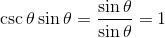and the right quantity simplifies such: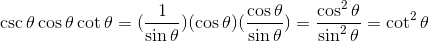Thus, we end up with: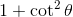,

which our Pythagorean identity tells us is equivalent to.

Thus,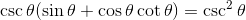Example Question #5 : Identities Of Squared Trigonometric Functions

Simplify using identities: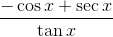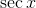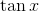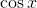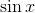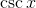Explanation:

First we expand the inverse identities into fractional form: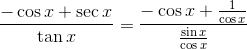Invert the bottom fraction and distribute into the top, keeping track of the negative: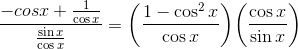Using the Pythagorean identity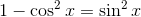, our equation becomes: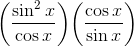At this point, cross-cancel to obtain.

Example Question #6 : Identities Of Squared Trigonometric Functions

Determine the value of: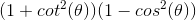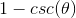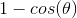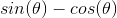Explanation:

In order to solve, first identify all the recognizable identities.

The identities that can be used for this scenario are: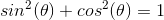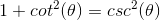The first identity can also be manipulated.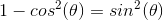Replacewith the correct identities and simplify.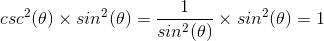Example Question #7 : Identities Of Squared Trigonometric Functions

Which of the following is equivalent to: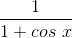?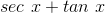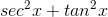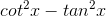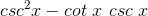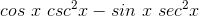Explanation:

We can multiply the bottom with its conjugate and obtain: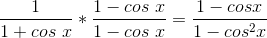Then we can use the pythagorean identity for the cosines and sines: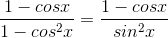Finally, we can split the fractions up and translate them into the trigonometric identity: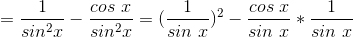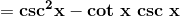Alternatively, you could take this and other answer choices and work the opposite way by translating all of the trigonometric ratios into sines and cosines, using the identities.

Another potential option is to try certain angle values for the original identity and selecting the choice that most closely matches the answer choices when the same angle is substituted (although one must beware of where the function(s) are undefined).